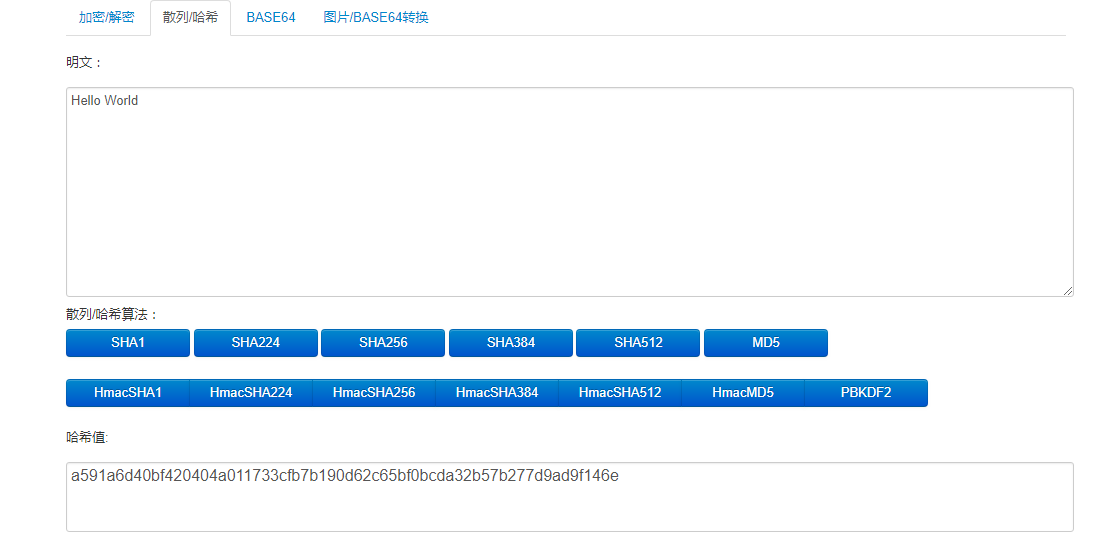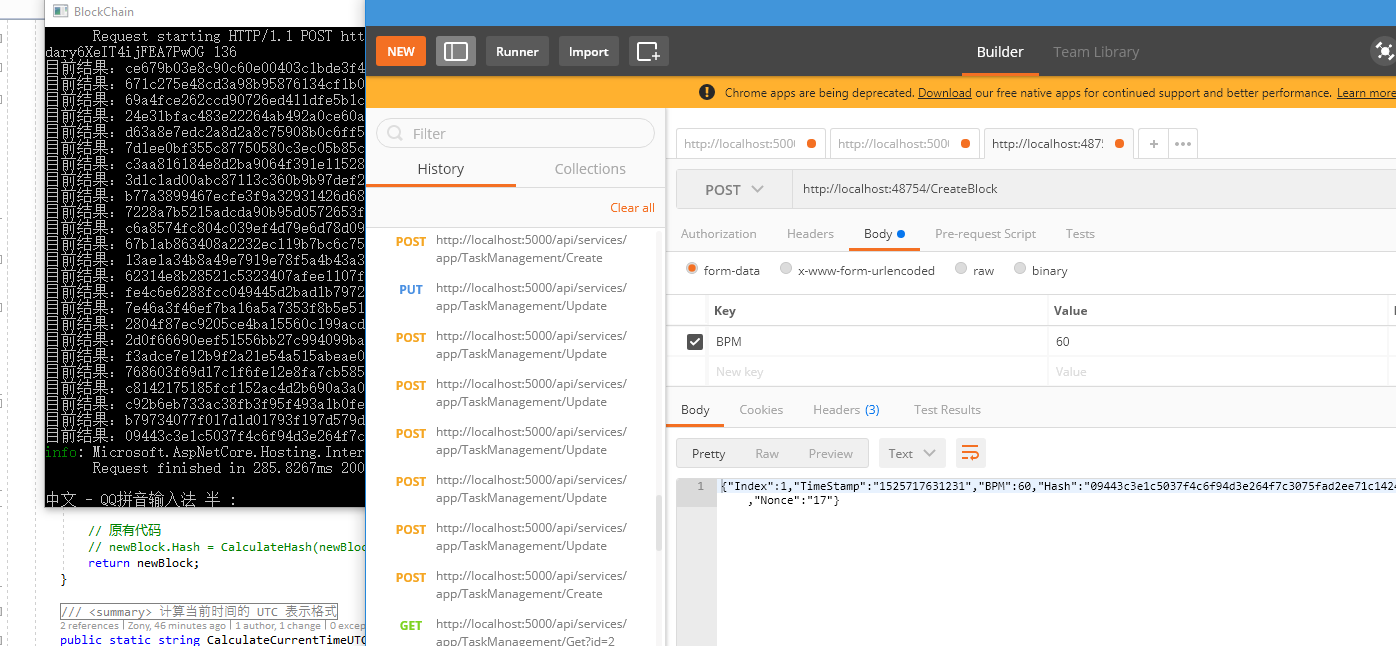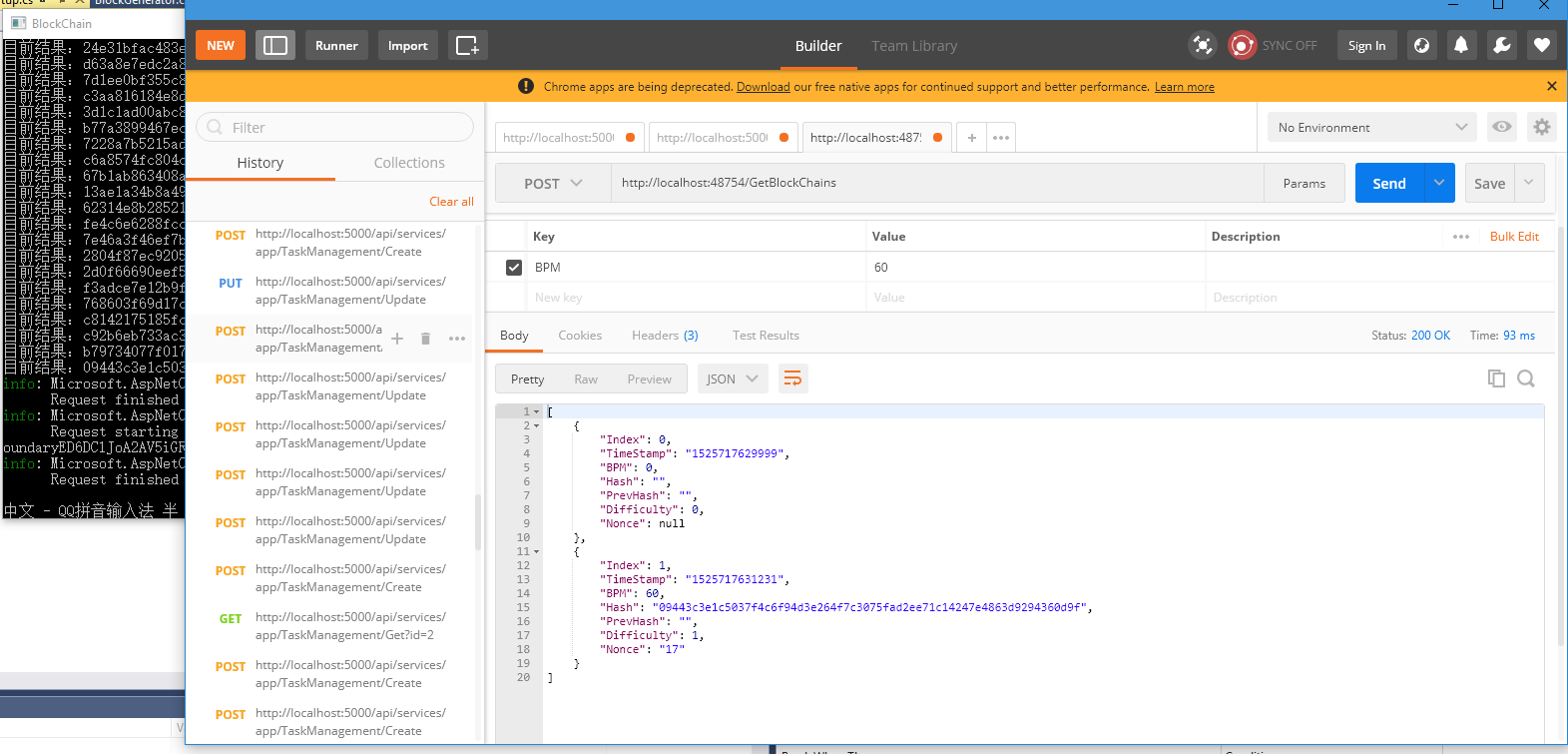# [C#] 使用 C# 编写自己区块链的挖矿算法

## 挖矿的原理

### 哈希/散列介绍## 工作证明

``````000f21ac06aceb9cdd0575e82d0d85fc39bed0a7a1d71970ba1641666a44f530
``````

## 动手开发

### 2.数据模型

``````public struct Block
{
/// <summary>
/// 区块位置
/// </summary>
public int Index { get; set; }
/// <summary>
/// 区块生成时间戳
/// </summary>
public string TimeStamp { get; set; }
/// <summary>
/// 心率数值
/// </summary>
public int BPM { get; set; }
/// <summary>
/// 区块 SHA-256 散列值
/// </summary>
public string Hash { get; set; }
/// <summary>
/// 前一个区块 SHA-256 散列值
/// </summary>
public string PrevHash { get; set; }
/// <summary>
/// 下一个区块生成难度
/// </summary>
public int Difficulty { get; set; }
/// <summary>
/// 随机值
/// </summary>
public string Nonce { get; set; }
}
``````

`Difficulty` 是一个整形，他定义了我们希望得到哈希前导 0 的数量，前导 0 越多，生成正确的散列值就越困难，我们现在从 1 开始。

`Nonce` 则是每次计算块散列值所需要的随机值。

### 3. 工作证明

``````/// <summary>
/// 校验 Hash 是否有效
/// </summary>
/// <param name="hashStr">Hash 值</param>
/// <param name="difficulty">难度</param>
/// <returns></returns>
public static bool IsHashValid(string hashStr, int difficulty)
{
var bytes = Enumerable.Range(0, hashStr.Length)
.Where(n => n % 2 == 0)
.Select(n => Convert.ToByte(hashStr.Substring(n, 2), 16))
.ToArray();

var bits = new BitArray(bytes);

for (var i = 0; i < difficulty; i++)
{
if (bits[i]) return false;
}

return true;
}
``````

``````/// <summary>
/// 计算区块 HASH 值
/// </summary>
/// <param name="block">区块实例</param>
/// <returns>计算完成的区块散列值</returns>
public static string CalculateHash(Block block)
{
string calculationStr = \$"{block.Index}{block.TimeStamp}{block.BPM}{block.PrevHash}{block.Nonce}";

SHA256 sha256Generator = SHA256.Create();
byte[] sha256HashBytes = sha256Generator.ComputeHash(Encoding.UTF8.GetBytes(calculationStr));

StringBuilder sha256StrBuilder = new StringBuilder();
foreach (byte @byte in sha256HashBytes)
{
sha256StrBuilder.Append(@byte.ToString("x2"));
}

return sha256StrBuilder.ToString();
}
``````

``````/// <summary>
/// 生成新的区块
/// </summary>
/// <param name="oldBlock">旧的区块数据</param>
/// <param name="BPM">心率</param>
/// <returns>新的区块</returns>
public static Block GenerateBlock(Block oldBlock, int BPM)
{
Block newBlock = new Block()
{
Index = oldBlock.Index + 1,
TimeStamp = CalculateCurrentTimeUTC(),
BPM = BPM,
PrevHash = oldBlock.Hash,
Difficulty = Difficulty
};

// 挖矿 ing...
for (int i = 0; ; i++)
{
newBlock.Nonce = i.ToString("x2");
if (!IsHashValid(CalculateHash(newBlock), Difficulty))
{
Console.WriteLine(\$"目前结果：{CalculateHash(newBlock)} ，正在计算中...");
continue;
}
else
{
Console.WriteLine(\$"目前结果：{CalculateHash(newBlock)} ，计算完毕...");
newBlock.Hash = CalculateHash(newBlock);
break;
}
}

// 原有代码
// newBlock.Hash = CalculateHash(newBlock);
return newBlock;
}
``````

## 效果